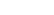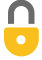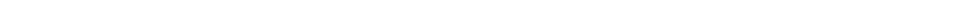Problem 1
Q

# How many significant figures do each of the following numbers have:

1. 214,
2. 81.60,
3. 7.03,
4. 0.03,
5. 0.0086,
6. 3236, and
7. 8700?A
a) $3$
b) $4$
c) $3$
d) $1$
e) $2$
f) $4$
g) $2$In order to watch this solution you need to have a subscription.VIDEO TRANSCRIPT

This is Giancoli Answers with Mr. Dychko. 214 has three significant figures and the number 81.60 has four significant figures; this zero is significant because there's no reason to put it there except to say this is a really precise number because 81.6 and 81.60 are the same quantity adding the zero is just an indication that this quantity is measured to this precision. And the number 7.03 has three significant figures. 0.03 has one significant figure, these zeros here don't count when you are talking about significant figures because they are just placeholder zeros; their only purpose is to put this number 3 where it belongs in the hundredths place. And the same kind of story applies to the next number 0.0086; it has two significant figures, only the 8 and the 6 are significant this 0.00 business here is just to put the 8 and the 6 in their proper place values. This number here has four significant figures and 8700 has two significant figures the way it's written here, this is a case that can sometimes be confusing, you might even see people say there's four significant figures but the best answer is two. If there was a little dot here, you might say that there's four because that would suggest that this number these two numbers were actually measured but the way it's written here, these zeros appear to serve only a placeholder function in that they make the 8 and the 7 go in the thousands and the hundreds place. So there's two significant figures here, only the 8 and the 7.

Giancoli Answers, including solutions and videos, is copyright © 2009-2023 Shaun Dychko, Vancouver, BC, Canada. Giancoli Answers is not affiliated with the textbook publisher. Book covers, titles, and author names appear for reference purposes only and are the property of their respective owners. Giancoli Answers is your best source for the 7th and 6th edition Giancoli physics solutions.